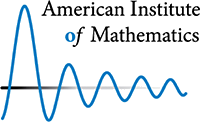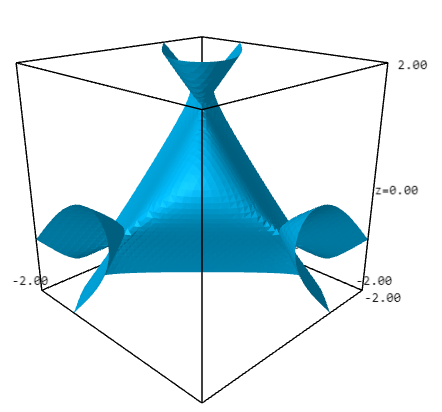### Highlights#### SQuaRE group mixes theory and computation

AIM - April 2022An ancient goal of mathematics is to classify objects based on their geometric properties.  The modern approach is to use techniques from another area of mathematics, often algebra, to solve what appears to be inherently a geometry problem.   Recent progress on manipulating systems of equations has vastly simplified that problem in many cases.  The research concerns Geometric Invariant Theory (GIT), which uses a system of equations to describe a landscape of geometric objects.  That is, each solution to the equations describes one object, and every object corresponds to one solution.  This enables one to use the tools of algebra to study a problem in geometry.  Now, having turned a geometry problem into an algebra problem, is the transformed problem easier?  Thanks to new research, it is -- in some cases.

The starting point of GIT is a system of equations, but that system will be redundant:  it will have extra symmetry which does not accurately reflect the underlying problem.  Removing that redundancy introduces a new problem:  some of the equations have undesirable properties which make the system difficult to analyze.  Those bad equations are called the "unstable locus".  Identifying and removing the unstable locus is the task which was automated by the SQuaRE group.  The work of the SQuaRE involved both devising a theoretical algorithm which can be used in a wide range of settings, and implementing that algorithm in computer code.

The implementation will be available, for free, for anyone to use.  More than a dozen recent research papers have used an ad-hoc approach to deal with special cases of this algorithm, often involving considerable effort.  The new computer code is able to do all of those previous calculations in a matter of minutes.  More significantly, the software and its underlying algorithm have been thoroughly tested, giving a high level of confidence in the result.  In addition to verifying previous work, the new code handles several new cases which were not accessible by previous methods.

The new software will facilitate the reproducibility of certain mathematical proofs -- the ability for a third party to check the results.  Reproducibility is fundamental to scientific research, including mathematics.  For traditional proofs the written words provide a complete argument, enabling a knowledgeable reader to verify that the proof is correct.  But if a portion of the proof involves a computer calculation, verification is difficult -- particularly so when the calculation involves specialized code.  The current work produced general code which applies in a multitude of cases.  This actually makes it easier to check that the code is correct, and thus makes it possible to verify the results in any specific case.

The algorithms have been implemented in Sagemath, a free and open source computer algebra system which serves as a fundamental tool for research in many areas of mathematics.  A paper describing the work is in the final stages of preparation.

This work was an outcome of the AIM SQuaRE "Computational aspects of GIT with a view of moduli spaces". SQuaRE participants were Patricio Gallardo (University of California, Riverside), Jesus Martinez-Garcia (University of Essex, UK), Han-Bom Moon (Fordham University), and David Swinarski (Fordham University).